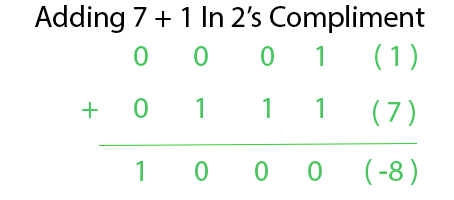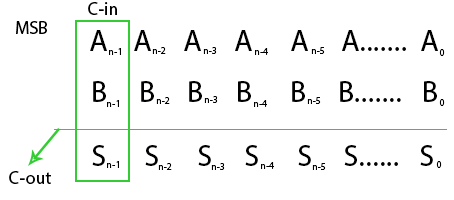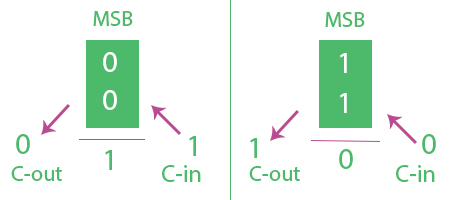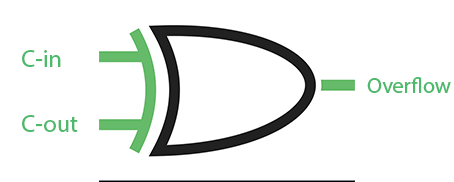# Overflow in Arithmetic Addition in Binary Number System

• Difficulty Level : Hard
• Last Updated : 15 Jul, 2021

In computer architecture 2’s Complement Number System is widely used. The discussion of overflow here mainly will we with respect to 2’s Complimentary System.

N-bit 2’s Complement number System can represent Number fromto4 Bit can represent numbers from ( -8 to 7 )
5 Bit can represent numbers from ( -16 to 15 ) in 2’s Complimentary System.

Attention reader! Don’t stop learning now.  Practice GATE exam well before the actual exam with the subject-wise and overall quizzes available in GATE Test Series Course.

Learn all GATE CS concepts with Free Live Classes on our youtube channel.

Overflow Occurs with respect to addition when 2 N-bit 2’s Complement Numbers are added and the answer is too large to fit into that N-bit Group.

A computer has N-Bit Fixed registers. Addition of two N-Bit Number will result in a max N+1 Bit number. That Extra Bit is stored in carry Flag. But Carry does not always indicate overflow.Adding 7 + 1 in 4-Bit must be equal to 8. But 8 cannot be represented with 4 bit 2’s complement number as it is out of range. Two Positive numbers were added and the answer we got is negative (-8). Here Carry is also 0. It is normally left to the programmer to detect overflow and deal with this situation.

Overflow Detection –
Overflow occurs when:

1. Two negative numbers are added and an answer comes positive or
2. Two positive numbers are added and an answer comes as negative.

So overflow can be detected by checking Most Significant Bit(MSB) of two operands and answer. But Instead of using 3-bit Comparator Overflow can also be detected using 2 Bit Comparator just by checking Carry-in(C-in) and Carry-Out(C-out) from MSB’s. Consider N-Bit Addition of 2’s Complement number.Overflow Occurs when C-inC-out. Above expression for overflow can be explained from below Analysis.In first Figure the MSB of two numbers are 0 which means they are positive. Here if C-in is 1 we get answer’s MSB as 1 means answer is negative (Overflow) and C-out as 0. C-inC-out hence overflow.

In second Figure the MSB of two numbers are 1 which means they are negative. Here if C-in is 0 we get answer MSB as 0 means answer is positive(Overflow) and C-out as 1. C-inC-out hence overflow.
Readers can also try out other combinations of c-in c-out and MSB’s to check overflow.

So Carry-in and Carry-out at MSB’s are enough to detect Overflow.Above XOR Gate can be used to detect overflow.

My Personal Notes arrow_drop_up